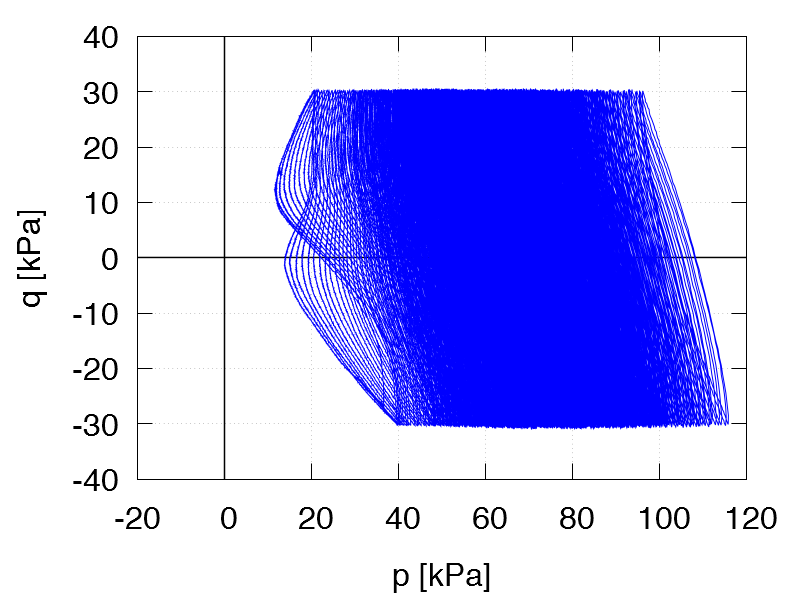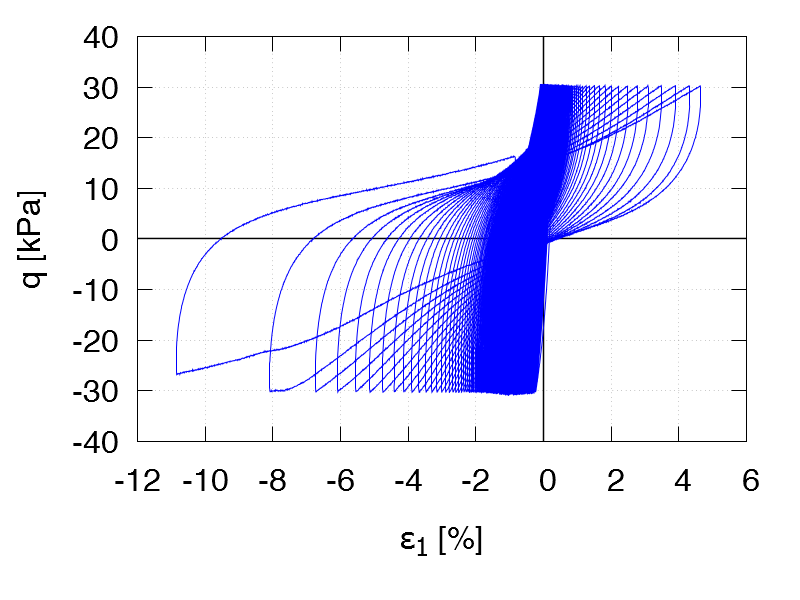Undrained cyclic tests with isotropic consolidation and stress cycles: Variation of overconsolidation ratio   Data of all tests of this series

All tests: p0 = 100 kPa, qampl = 30 kPa, Displacement rate 0.1 mm/min
Test C37
e0 = 1.239
OCR = 1.5

Test data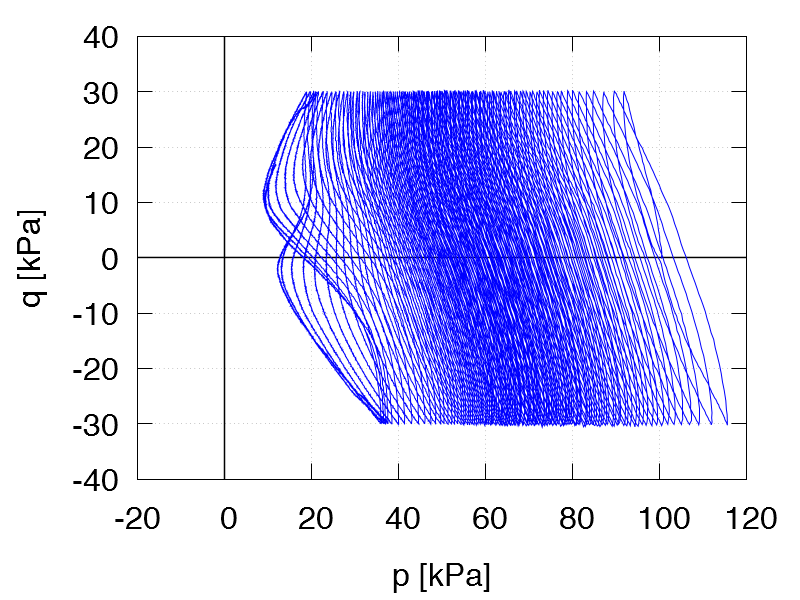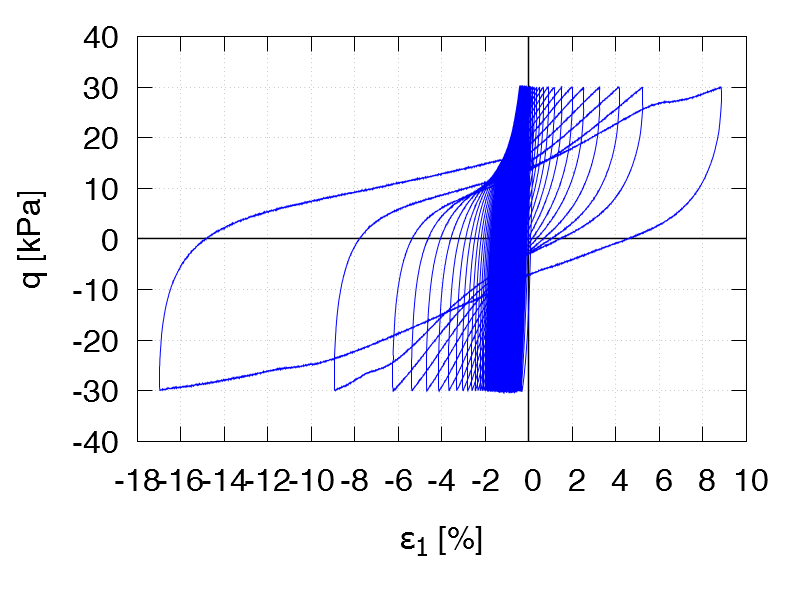Test C38
e0 = 1.200
OCR = 2.0

Test data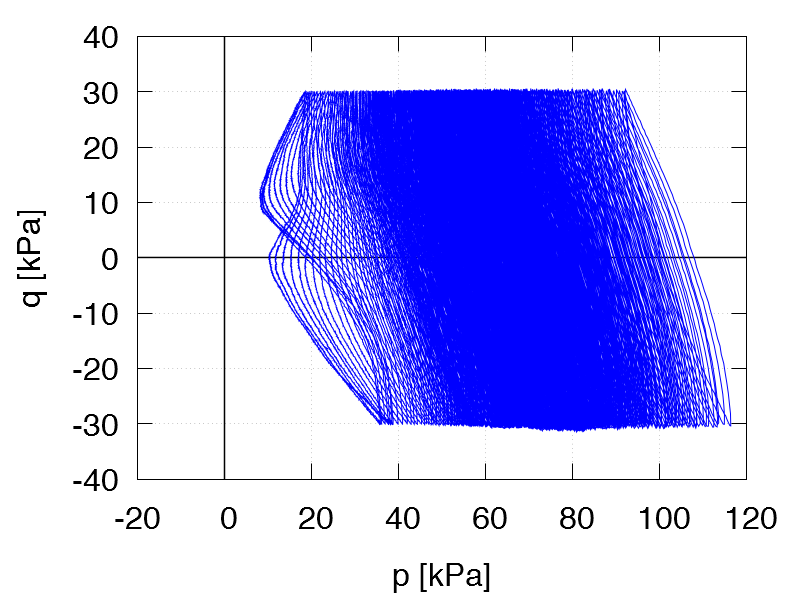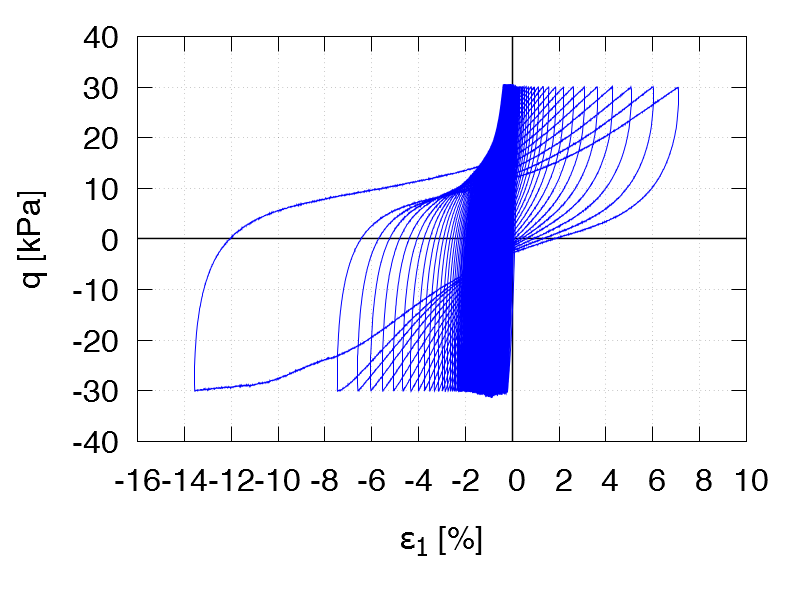Test C39
e0 = 1.146
OCR = 2.5

Test data